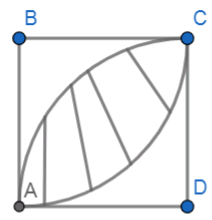Courses
Courses for Kids
Free study material
Free LIVE classes
MoreLIVE
Join Vedantu’s FREE Mastercalss

# In the diagram, a square ABCD has a side with a length of 6cm. Circular arcs of radius 6cm are drawn with centres B and D. What is the area of the shaped region in sq.cm?Verified
359.4k+ views
Hint: In this question, first draw the diagram of the square and then draw the circular arcs of given radius. So, it gives a clear picture of what we have to find. Now, try to form an equation for the required area using the area of the square and area of the circular arc.

Let us look at some of the definitions and formulae.
SQUARE: In geometry, a square is a regular quadrilateral, which means that it has four equal sides and four equal angles. It can also be defined as a rectangle in which two adjacent sides have equal length. Hence, the diagonals are equal and they bisect at right angles.
CIRCULAR ARC: An arc is a segment of a circle. A common curved example is an arc of a circle, called a circular arc.
In general, the area is defined as the region occupied inside the boundary of a flat object or figure.
Area of a square of side length s is given by:
$A={{s}^{2}}$
Area of a circular arc of radius r is given by:
$A=\dfrac{1}{2}{{r}^{2}}\varphi$
Where r is the radius of the circular arc and $\varphi$ is the angle subtended by the arc at the centre.
Now, let us draw the diagram with the given conditions.
Given,
AB = BC = CD = DA = 6cm
Radius of the circular arcs = 6cmNow, the area of the shaded region can be found by finding the area of the square and the area of the circular arc.
Let us assume the area of the square as A1 and area of the circular as A2.
\begin{align} & \Rightarrow {{A}_{1}}={{s}^{2}} \\ & \Rightarrow {{A}_{1}}={{6}^{2}}\text{ }\left[ \because s=6 \right] \\ & \therefore {{A}_{1}}=36c{{m}^{2}} \\ \end{align}
\begin{align} & \Rightarrow {{A}_{2}}=\dfrac{1}{2}{{r}^{2}}\varphi \\ & \Rightarrow {{A}_{2}}=\dfrac{1}{2}{{6}^{2}}\dfrac{\pi }{2}\text{ }\left[ \because r=6,\varphi =\dfrac{\pi }{2} \right] \\ \end{align}
Now, on simplification we get,
\begin{align} & \Rightarrow {{A}_{2}}=\dfrac{36}{4}\pi \\ & \therefore {{A}_{2}}=9\pi c{{m}^{2}} \\ \end{align}
Let us assume that the area of the shaded region as A3.
Now, on writing A3 in terms of A1 and A2 we get,
$\Rightarrow {{A}_{3}}=2\left( {{A}_{2}}-\dfrac{{{A}_{1}}}{2} \right)$
By applying the respective values in the above equation we get,
\begin{align} & \Rightarrow {{A}_{3}}=2\left( 9\pi -\dfrac{36}{2} \right) \\ & \Rightarrow {{A}_{3}}=2\left( 9\pi -18 \right) \\ & \therefore {{A}_{3}}=18\left( \pi -2 \right)c{{m}^{2}} \\ \end{align}

Note:
Instead of using the formula for area of the square and area of the circular arc we can alternatively find the area of the shaded region by using applications of integration.
In order to find the area of shaded region using applications of integration we first need to know the equation of the curves and then integrate them within the proper limits using the formula:
$\int\limits_{a}^{b}{f\left( x \right)-g\left( x \right)dx}$
While calculating the area of the shaded region it is important to note that as given figure is a square so area will be halved in which subtracting the area of half of the square from the area of one circular arc gives only half of the area of the shaded region so we need to multiply it with 2.

Last updated date: 20th Sep 2023
Total views: 359.4k
Views today: 5.59k